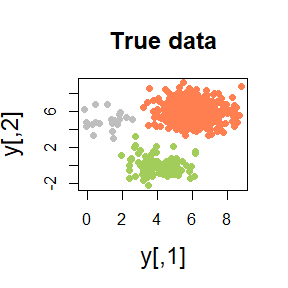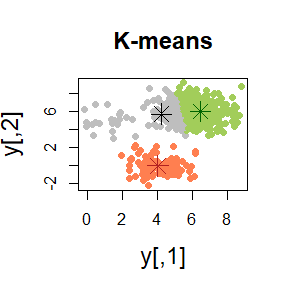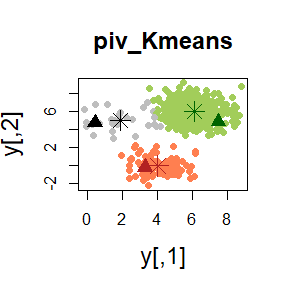# K-means clustering using MUS and other pivotal algorithms

#### 2019-06-24

In this vignette we explore the K-means algorithm performed using the MUS algorithm and other pivotal methods through the function piv_KMeans of the pivmet package. First of all, we load the package:

library(pivmet)

## Pivotal algorithms: how they works, and why

We present here a simulated case for applying our procedure. Given $$n$$ units $$y_1,\ldots,y_n$$:

• consider to build a co-association matrix $$C$$, by taking the co-occurrences of pairs of $$n$$ units in the same cluster/group among the total number of partitions. For instance, this matrix could be constructed from a MCMC output arising from Bayesian mixture models;

• suppose we want to detect the pivotal units, as the observations that are as far away from each other as possible according to the co-association matrix. Units which are very distant from each other are likely to have zero co-occurrences;

• the resulting units—hereafter pivots—have the desirable property to be representative of the group they belong to.

We propose four alternative methods for achieving this task. Let $$j, \ j=1,\ldots,k$$ be the group containing units $$\mathcal J_j$$, the user may choose $${i^*}\in\mathcal J_j$$ that maximizes one of the quantities:

\begin{align*} & \sum_{p\in\mathcal J_j} c_{{i^*}p} \\ & \sum_{p\in\mathcal J_j} c_{{i^*}p} - \sum_{p\not\in\mathcal J_j} c_{{i^*}p}. \end{align*}

These methods give the unit that maximizes the global within similarity (maxsumint) and the unit that maximizes the difference between global within and between similarities (maxsumdiff), respectively. Alternatively, we may choose $$i^{*} \in\mathcal J_j$$, which minimizes:

$\sum_{p\not\in\mathcal J_j} c_{i^{*}p},$

obtaining the most distant unit among the members that minimize the global dissimilarity between one group and all the others (minsumnoint).

MUS algorithm described in Egidi et al. (2018b) is a sequential procedure for extracting identity submatrices of small rank and pivotal units from large and sparse matrices. The procedure has already been satisfactorily applied for solving the label switching problem in Bayesian mixture models (Egidi et al. 2018c).

With the function MUS the user may detect pivotal units from a co-association matrix C, obtained through $$H$$ different partitions, whose units may belong to $$k$$ groups, expressed by the argument clusters. We remark here that MUS algorithm may be performed only when $$k <5$$.

#generate some data

set.seed(123)
n  <- 620
centers  <- 3
n1 <- 20
n2 <- 100
n3 <- 500
x  <- matrix(NA, n,2)
truegroup <- c( rep(1,n1), rep(2, n2), rep(3, n3))

x[1:n1,]=rmvnorm(n1, c(1,5), sigma=diag(2))
x[(n1+1):(n1+n2),]=rmvnorm(n2, c(4,0), sigma=diag(2))
x[(n1+n2+1):(n1+n2+n3),]=rmvnorm(n3,c(6,6),sigma=diag(2))

H <- 1000
a <- matrix(NA, H, n)

for (h in 1:H){
a[h,] <- kmeans(x,centers)$cluster } #build the similarity matrix sim_matr <- matrix(1, n,n) for (i in 1:(n-1)){ for (j in (i+1):n){ sim_matr[i,j] <- sum(a[,i]==a[,j])/H sim_matr[j,i] <- sim_matr[i,j] } } cl <- KMeans(x, centers)$cluster
mus_alg <- MUS(C = sim_matr, clusters = cl, prec_par = 5)

## piv_KMeans: k-means clustering via pivotal units

In some situations, classical K-means fails in recognizing the true groups:For instance, when the groups are unbalanced or non-spherical shaped, we may need a more robust version of the classical K-means. The pivotal units may be used as initial seeds for K-means method (Egidi et al. 2018a). The function piv_KMeans works as the kmeans function, with some optional arguments

piv_res <- piv_KMeans(x, centers)The function piv_KMeans has optional arguments:

• alg.type: The clustering algorithm for the initial partition of the $$N$$ units into the desired number of clusters. Possible choices are "KMeans" (default) and "hclust".

• method: If alg.type is "hclust", the character string defining the clustering method. The methods implemented are "single","complete", "average", "ward.D", "ward.D2", "mcquitty","median", "centroid". The default is "average".

• piv.criterion: one among the four different pivotal criteria described above and listed in Egidi et al. (2018c): MUS, maxsumint, minsumnoint, maxsumdiff. MUS is the default criterion when centers $$\le$$ 4, whereas maxsumint is the default method when centers > 4.

• H: the number of distinct $$k$$-means runs used for building the $$N \times N$$ co-association matrix. Default is $$10^3$$.

• iter.max: if alg.type is "KMeans", the maximum number of iterations to be passed to KMeans(). Default is 10.

• num.seeds: If alg.type is "KMeans", the number of different starting random seeds to be passed to KMeans(). Default is 10.

• prec_par: with this argument the user may increase the powerful of the underlying MUS algorithm (see Egidi et al. (2018b) for details). The usual choice is $$\min\{ 10, \underset{j}{\min}n_j \}$$, where $$n_j$$ is the number of units belonging to the group $$j, \ j=1,\ldots,k$$.